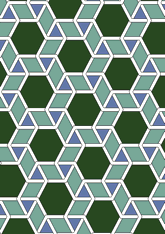# Mosque of ibn Tulundata188/EGY0511

## Geometry

• The symmetry group of the tiling is 632 (p6).
• All the internal angles of the constituent polygons are a multiple of 60°.
• Contains one equilateral triangle.
• Contains one regular two-pointed star polygon with vertex angle of 60°.
• Contains one regular hexagon.
• The tiling satisfies the two-colour condition.
• The tiling is edge-to-edge.
• As drawn, contains about 179 polygons.

## References

Publications referenced: